Interactive ICSE S Chand Maths Class 9 Solutions Chapter 18 Surface Area and Volume of 3D Solids Ex 18(B) engage students in active learning and exploration.

## S Chand Class 9 ICSE Maths Solutions Chapter 18 Surface Area and Volume of 3D Solids Ex 18(B)

Question 1.
Find the volume of a rail of uniform crosssection, given area of cross-section 12.8 cm2, and length 1.26 cm.
Solution:
Area of the cross-section of a rail = 12.8 cm2 and length = 1.26 cm
∴ Volume = area × length =12.8 × 1.26 cm3 = 16.128 cm3

Question 2.
Find the area of the cross-section, assuming it to be uniform, of a solid, given that its volume is 92.8 cm3, and length is 6.4 m.
Solution:
Volume of a cross-section of a solid = 92.8 cm3
and its length = 6.4 m = 640 cm
∴ Area of the cross-section = $$\frac{\text { Volume }}{\text { Length }}$$
= $$\frac{92.8}{6.40}$$ cm2 = 0.145 cm2Question 3.
The dimensions of the L-shaped crosssection of bar, 2 m long, are shown in cm in figure. Find
(i) the volume of the bar.
(ii) the weight, if the material weighs 0.3 kg per cm3.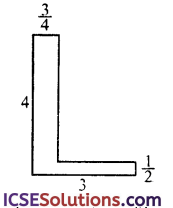Solution:
Length of cross-section of bar = 2 m = 200 cm
In the figure produced DC which meets AF at G
Now AG = 4 – $$\frac{1}{2}$$ = 3$$\frac{1}{2}$$ cm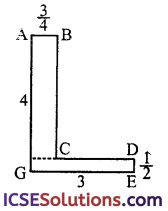(i) ∵ Area of the figure of the cross-section = Area of ABCD + Area of GDEF

Area of ABCG = 3$$\frac{1}{2}$$ × $$\frac{3}{4}$$ =$$\frac{7}{2}$$ × $$\frac{3}{4}$$ = $$\frac{21}{8}$$cm2
∴ Total area = $$\frac{21}{8}$$ + $$\frac{3}{2}$$ = $$\frac{21+12}{8}$$ = $$\frac{33}{8}$$ cm2
∴ Volume of the cross-section = Area × Length
= $$\frac{33}{8}$$ × 200 = 33 × 25 cm3 = 825 cm3

(ii) Weight of 1 cm3 = 0.3 kg
∴ Total weight = 825 × 0.3 kg = 247.5 kg

Question 4.
The dimensions of the cross-section of a girder, 2.5 m long, are shown in cm in the diagram. Find
(i) the volume of the girder,
(ii) the weight, if the material weighs 7.8 gm per cm2.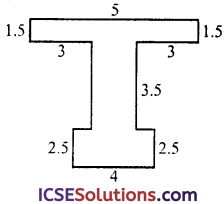Solution:
Length of cross section = 2.5 m = 250 cm In the given figure,On joining LM and JK, we get three rectangles
∴ Area of rectangle I
=4 × 2.5 = 10 cm2

Area of rectangle II = 3.5 × (8 – 3 – 3)
= 3.5 × 2 = 7 cm2
and area of rectangle III = 8 × 1.5 = 12 cm2
∴ Total area of the cross section = 10 + 7 + 12 = 29 cm2

(i) ∴ Volume = Area × Length
= 29 × 250 = 7250 cm3

(ii) Weight of 1 cm3 = 7.8 gm
∴ Total weight = 7.8 × 7250 gm = 56550 gm = 56.55 kg = 57 kg (approx)

Question 5.
The cross-area of a pipe is 42 cm2, and water is pouring out of it at the rate of 1.25 m per sec. If the pipe remains full, find the number of litres discharged per minute.
Solution:
Area of cross-section of a pipe = 42 cm2
= $$\frac{42}{100 \times 100}$$ m2

Rate of water pouring = 1.25 m per sec
Rate of water per minute = 1.25 m × 60 = 75.00 m per minute
∴ Volume of water in the pipe

= $$\frac{42}{100 \times 100}$$ × 75 = 0.315 m3
Water in litres = 0.315 × 1000
= 315 litres (1 m2 = 1000 l)

Question 6.
The figure shows a solid of uniform crosssection. Find the volume of the solid. All measurements are in centimetres. Assume that all angles in the figure are right angles.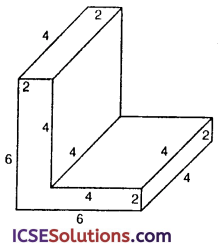Solution:
Length of the cross-section = 4 c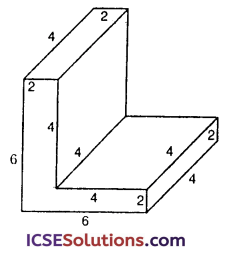Area of the cross-section = 4 × 2 + 6 × 2
= 8 + 12 = 20 cm2
∴ Volume = Area × Length
= 20 × 4 = 80 cm3Question 7.
A swimming pool is 50 m long and 15 m wide. Its shallow and deep ends are 1$$\frac{1}{2}$$ m and 4$$\frac{21}{8}$$ m deep respectively. If the bottom of the pool slopes uniformly, find the amount of water in litres required to fill the pool.
Solution:
Length of swimming pool (l) = 50 m and width (b) = 15 m

Depth of water in one side = 1$$\frac{1}{2}$$ m
and other side = 4$$\frac{1}{2}$$ m
∴ Mean depth (h) = $$\frac{1}{2}$$ $$\left[1 \frac{1}{2}+4 \frac{1}{2}\right]$$m
= $$\frac{1}{2}$$ × 6 = 3 m
∴ Volume of the pool = lbh
= 50 + 15 × 3 = 2250 m3
∴ Volume of water in litres = 2250 × 1000 l (1 m3 = 1000 l) = 2250000 litres

Question 8.
The area of cross-section of a pipe is 5.4 cm2 and water is pumped out of it the rate of 27 km / h. Find in litres the volume of water which flows out of the pipe in one minute.
Solution:
Area of the cross section = 5.4 cm2 Flows of water = 27 km / h
Flow of water in 1 minute = $$\frac{27 \times 1000}{60}$$m = 450 m
∴ Volume of the water = $$\frac{5.4}{100 \times 100}$$ × 450 m3 = 6.243 m3
∴ Water in litres = 0.243 × 1000 litres (1 m3 = 1000 l) = 243 litres

Question 9.
The horizontal cross-section of a water tank is in the shape of a rectangle with a semicircle at one end, as shown in figure. The water is 2.4 m deep in the tank. Calculate the volume of water in the tank in gallons.
( Take π to be $$\frac{22}{7}$$ and 1 gallon to be 4.5 litres)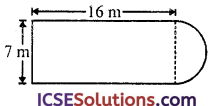Solution:
Area of the cross-section of the tank = area of rectangle ABCD + area of semicircle or BC= l × b + $$\frac{1}{2}$$πr2
= 16 × 7 + $$\frac{1}{2}$$ × $$\frac{22}{7}$$ × $$\frac{7}{2}$$ × $$\frac{7}{2}$$ m2 $$\left(r=\frac{7}{2} \mathrm{~m}\right)$$
= 112 + $$\frac{77}{4}$$ = $$\frac{448+77}{4}$$ = $$\frac{525}{4}$$ m2
Depth of water in the tank = 2.4 m
∴ Volume of water = area × depth
= $$\frac{525}{4}$$ × 2.4 m3 = 315 m3
Water in gallons = 315 × 1000 l=$$\frac{315 \times 1000}{4.5}$$ gallons
& =70000 gallon

Question 10.
In the figure, shows the end face of a triangular girder which is 5 m long. Find the volume of metal used in casting it. (Units in cm).Solution:
Cross-section of the girder is triangular whose base (b) = 60 m and height (h) = 90 cm
∴ Area of the face of the girder
= $$\frac{1}{2}$$ × b × h = $$\frac{1}{2}$$ × 60 × 90 cm2 = 2700 cm2
Length of girder = 5 m
∴ Volume of metal used
= Area × Length
= $$\frac{2700 \times 5}{100 \times 100}$$ m3
= $$\frac { 135 }{ 100 }$$ m3 = 1.35 m3

Question 11.
In the figure shows the end-view of a swimming pool which is 10 m wide. Calculate the volume of water when it is full of water $$\frac { 1 }{ 2 }$$ m from the top.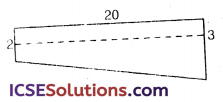Solution:
Width of pool = 10 m
Water = $$\frac { 1 }{ 2 }$$ m from the top
Length = 20 m
∴ Height of water from one side = 2 – $$\frac { 1 }{ 2 }$$
= 1$$\frac { 1 }{ 2 }$$ m
∴ Average height = $$\frac { 1 }{ 2 }$$ $$\left(1 \frac{1}{2}+2 \frac{1}{2}\right)$$
= $$\frac { 1 }{ 2 }$$ × 4 = 2 m
∴ Volume of water in the pool = l × b × h
= 20 × 10 × 2 m3 = 400 m3Question 12.
In the figure shows the end-face of a garage which is 20 m long.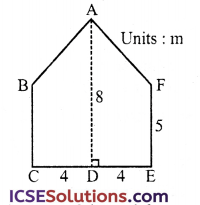(i) Find the area of the end-face.
(ii) Find the volume of air-space of the garage in m3.
(iii) If 40 m3 of air is required per worker, how many workers can be employed in this garage ?
Solution:
Length of garage = 20 m
By joining BF, we get the end face of the garage, one rectangle and one triangle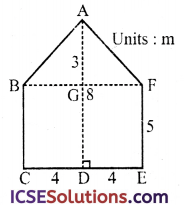∴ Area of rectangle BCEF = CE × BC = 8 × 5 = 40 m2
and area of triangle ABF
= $$\frac { 1 }{ 2 }$$ BF × AG
= $$\frac { 1 }{ 2 }$$ × 8 × 3 = 12 m2

(i) ∴ Total area of the end-face = 40 + 12 = 52 m2
(ii) Volume of the air space = area × length = 52 × 20 = 1040 m3
(iii) For one worker air is required = 40 m3
∴ No. of workers = $$\frac { 1040 }{ 40 }$$ = 26